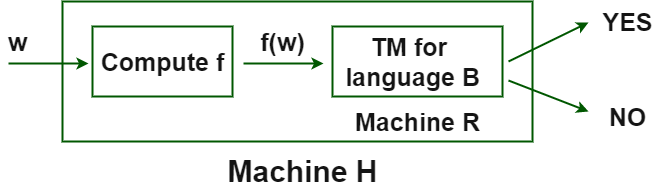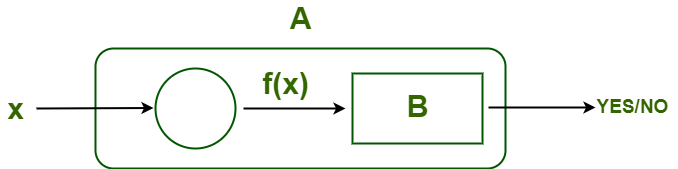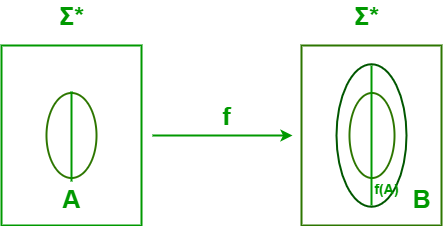Open in App
Not now

# Reduction Theorem in TOC

• Difficulty Level : Expert
• Last Updated : 10 Sep, 2021

Reduction Theorem :
A reduction from A to B is a function

`                          f : Σ1* → Σ2* such that For any w ∈ Σ1*, w ∈ A if f(w) ∈ B`H accepts w if R accepts f(w) if f(w)∈ B if w ∈ A

• Every w ∈ A maps to some f(w) ∈ B.
• Every w ∉ A maps to some f(w) ∉ B.
• f does not have to be injective or surjective.

Why Reductions Matter?
If language A reduces to language B, we can use a recognizer / co-recognizer / decider for B to recognize / co-recognize / decide problem A.

If A is reducible to B ( A<= B ) –

• Problem A is easily reducible to Problem B that clearly states that – the problem ‘B’ is at least as hard as problem ‘A’.OR

• ∀x, x ∈ A, if f(x) ∈ B; where f is many to one reduction from A to B, which is denoted as ( A <=m B ).1. A <= B – Problem A is reducible to problem B.
2. A <=m B – Problem A is many to one reducible to problem B.
3. A <=m B – Problem A is reducible in polynomial manner to problem B.

Mapping Reductions :

• A function f : Σ1* → Σ2* is called a mapping reduction from A to B if For any w ∈ Σ1*, w ∈ A if(w) ∈ B.
• f is a computable function.
• Intuitively, a mapping reduction from A to B says that a computer can transform any instance of A into an instance of B such that the answer to B is the answer to A.

Properties of Reduction :

• If A<=B, and A is undecidable then B is also undecidable.
• If A<=B, and B is undecidable then A need not to be undecidable.
• If A<=B, and A is decidable then B need not to be undecidable.
• If A<=B, and B is decidable then A is also decidable.
• If A<=B, and B is recursive then A is also recursive.
• If A<=B, and A is recursive then B need not to be recursive.
• If A<=B, and B is recursive enumerable then A is also recursive enumerable.
• If A<=B, and A is recursive enumerable then B need not to be recursive enumerable.
• If A<=B, and B is P-problem then A is also P-problem.
• If A<=B, and A is P-problem then B need not to be P-problem.
• If A<=B, and B is NP-problem then A is also NP-problem.
• If A<=B, and A is P-problem then B need not to be P-problem.
• If A<=B and B<=P then A<=P (transitivity).
• If A<=B and B<=A then A and B are polynomial equivalent.
• If A<=B and A is not REL then B is also not REL.
• If A<=B, and A is not P-problem then B is also not P-problem.
• If A<=B and A is not recursive problem then B is also not recursive problem.

Examples –

1. A :  t4 – 1 ————- B : t2 – 1 , C : t2 + 1
In example 1 since A is solvable and B,C<A so, B and
C is also solvable

` since, ( t4 - 1 )= ( t2 - 1 ) * ( C : t2 + 1 ) `

2. A :  Is L(D) = Σ* ? ———> Problem A can be reduced to B : Is L(D1) = Σ* – L(D2)
In example B is subset of A so A is reduced to Problem B.

3. A :  Is L(G) = NULL ? ———> Problem A can be reduced to B : Is L(G1) is subset of L(G2) ?
If above problem A is reduced to a simpler form problem B then solution would be easy.

```4. A :  a3 + b3 + 3a2b + 3b2a --------------> A is reduced to B : ( a + b )3
If A reduces to B and B is “solvable,” then A is “solvable.” ```

5. Reduction of LD to  ————->  0* 1*
Wyes =01  and  Wno= 10
Then reduced form f(w) will be :  f (w)={ 01  if w ∈ LD  ,  10 if  w ∉ LD }

6. Find reduced grammar equivalent to grammar G, having production rules

`P : S --> AC | B, A --> a, C --> c | BC, E --> aA | e`
``` Phase 1 - T= {a ,c, e},  W1= {A,C,E},   W2= {A,C,E,S},  W3= {A,C,E,S}
G' = { (A,C,E,S), (A,C,E), P, (s) },
P: S --> AC , A --> a, C --> c, E --> aA | e
Phase 2 -  Y1= {S}  ,  Y2 = {S,A,C}  ,  Y3= {S, A, C, a, c}  ,  YL1 = { S, A, C, a, c }
G'' = { (A,C,S), (a,c), P, {S} },
P: S --> AC , A --> a, C --> c```

7. Problem A (Hard Problem) – Move from New Guinea to Amazon City.
( Since, we know their is an easy way from New Guinea to Canada) So, we will reduce the problem into easier one.

Problem B(easier problem) – Move from Canada to Amazon City.
So, It is clear if we can find a solution to the easier problem, then we can use it to solve a harder one.

8.  Given  Problem A : L1<=L2 and L2<=L3

1. If L2 is decidable then —–> L1 is decidable  and  L3 is either decidable or not decidable.
2. If L2 is undecidable then —–> L1 is either decidable or not decidable  and  L3 is undecidable .
My Personal Notes arrow_drop_up